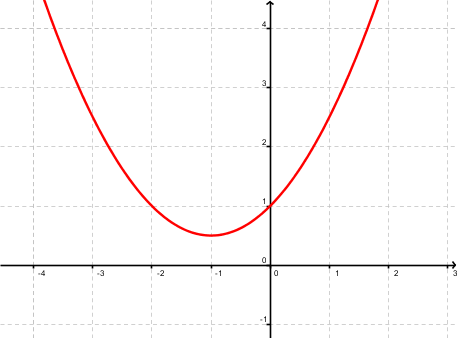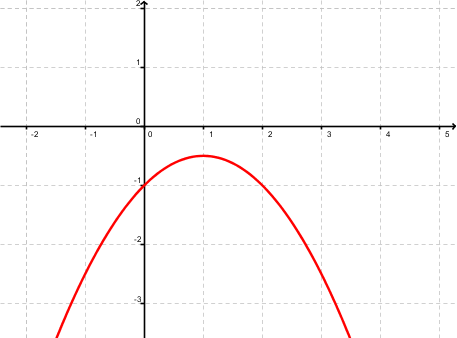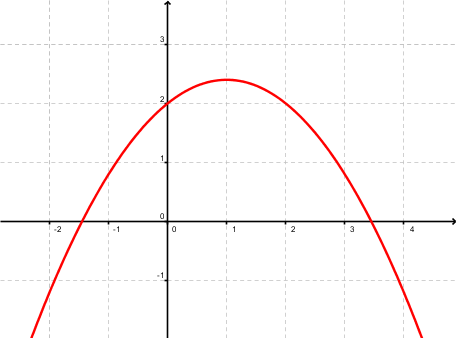Math Calculators, Lessons and Formulas

It is time to solve your math problem

mathportal.org
• Quadratic Equations, Inequalities, and Functions
• Discriminant of a quadratic equation

# Discriminant of a quadratic equation

ans:
syntax error
C
DEL
ANS
±
(
)
÷
×
7
8
9
4
5
6
+
1
2
3
=
0
.
auto next question
calculator
•  Question 1: 1 pts Discriminant of the quadratic polynomial $ax^2 + bx + c$ is $$D=b^2-4ac$$
•  Question 2: 1 pts The discriminant of quadratic equation $x^2-3x+2=0$ is:
 $1$ $2$ $3$ $4$
•  Question 3: 1 pts The discriminant of quadratic equation $2x^2-3=0$ is:
 $24$ $-24$ $12$ $-12$
•  Question 4: 1 pts The discriminant of quadratic equation $x^2=0$ is:
 $-1$ $0$ $1$ $2$
•  Question 5: 1 pts If the discriminant of an equation is $> 0$, which of the following is true
 Equation has two complex solutions Equation has one real solution Equation has two real solutions
•  Question 6: 2 pts Let $D$ be a discriminant of the quadratic equation which graph is shown at the right. Which of the following statements is true?$D>0$ $D<0$ $D=0$
•  Question 7: 2 pts If the discriminant is a perfect square the roots are rational.
•  Question 8: 2 pts How many and what type of solutions would an equation have if the discriminant is 1234?
 two complex solutions one real solution two real solutions
•  Question 9: 2 pts State the nature of the roots of the following quadratic equations $2x^2-5x+4=0$
 two complex solutions one real solution two real solutions
•  Question 10: 3 pts Let $D$ be a discriminant of the quadratic function $f(x)=ax^2+bx+c$ , which graph is shown at the right. Which of the following statements is true?a>0 , D>0 a<0 , D<0 a>0 , D<0 a<0 , D>0
•  Question 11: 3 pts Let $D$ be a discriminant of the quadratic function $f(x)=ax^2+bx+c$ , which graph is shown at the right. Which of the following statements is true?$a<0 , D>0 , c>0$ $a>0 , D>0 , c<0$ $a<0 , D<0 , c>0$
•  Question 12: 3 pts If the value of a discriminant is a perfect square, then the quadratic polynomial can be factored.
•  Question 13: 3 pts If the value of a discriminant is zero, then the quadratic polynomial is a perfect square.
•  Question 14: 3 pts Determine $k$ so that $x^2-10x+k=0$ has two equal real solutions.
 $10$ $15$ $20$ $25$
•  Question 15: 3 pts Determine $k$ so that $kx^2-2kx+1=0$ has two equal real solutions.
 $k = 1 \, or\, k = 0$ $k = 0$ $k = 1$ $k = 2 \,or \, k = 0$